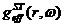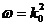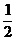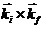## Wednesday 21 August 2019

### Physics of Nucleon-Nucleus Scattering - Chapter IIC

The effective NN interactions

Coordinate space effective NN interactions, between protons with energies to over 300 MeV incident upon nuclei and each bound nucleon in the target, have been defined by optimal fits to the on- and half-off-shell t and nuclear matter g matrices that have been determined from solutions of the LS and BBG equations respectively. Realistic NN potentials, such as Paris , Bonn-B , and OSBEP  have been used as input. Folding these medium modified effective interactions with appropriate density matrices give nonlocal optical potentials for the elastic scattering of protons from nuclei. As will be shown, good reproduction of the differential cross section data and diverse spin observables with scattering from all nuclei in the range 3He to 238U has been obtained [74-78] by solving the associated nonlocal Schrodinger equations.

Herein, a specific form of the effective interaction in coordinate space that is useful in making a folding optical potential is specified.  That form of the effective interaction is dictated by the structure used in the programs DWBA91 and DWBA98 [30, 31].  Not only do those programs evaluate the nonlocal optical potentials from a full folding prescription but also they solve the associated integro-differential Schrodinger equations for pA scattering.

Coordinate space representations

Momentum space studies of NN scattering below pion threshold involve solution of partial wave LS equations starting with one of the realistic interactions. The result is a tabulation of arrays

for each on-shell energy w.  But the spin structure of the t matrix is very important, so classification in terms of central, tensor, and two-body spin-orbit characteristics has proven useful .  Also, meson exchange concepts, when used to define the NN interactions, often are more conveniently described in coordinate space with Yukawa form factors. A number of the interactions such as the Reid , the Paris [20, 21], and the Bonn-R  forces were determined in this way. Further, if one wishes to consider more complicated NA systems and their reactions, such a representation has been used for most studies that go beyond the simplest of microscopic calculations [29, 80]. Indeed, to obtain sensible optical model potentials medium corrections must be considered.  Only by so doing can one obtain reasonable predictions of differential cross sections and spin observables from proton-nucleus elastic scattering for energies to 300 MeV and above.

There are circumstances in which interactions do not have convenient structure.  Interactions which necessarily have been obtained solely from the NN scattering data   are examples.  In such cases, an effective parameterization scheme facilitates a recasting of the t matrices into utilitarian nuclear operator structure.  One such effective interaction scheme consists of central (C), tensor (S12), and two-body spin-orbit (L.S) components each of which has a form factor that is a set of Yukawas of various ranges.  Each of those Yukawas has a complex strength which is dependent upon the energy and the spin/isospin state.

The parameterization scheme

The form of an effective interaction in coordinate space that is now used is

, ........................................(2.39)

where the radial functions have the form,

, ....................................(2.40)

for each operator of the setare the inverse ranges of the interaction, and j represents the set of the inverse ranges chosen. In principle, the number of strengths and ranges chosen can be as large as one likes, though experience shows that four suffice with all operators to accurately reproduce the half-off-shell g-matrices for energies between 25 and 800 MeV.

The basic `data', the g-matrix elements are in JSTLL' channel form and these have to mapped against effective interaction elements in ST channel form. To do so, the angular momentum state expectation values of the operators in the effective interactions are needed. Explicitly, those expectation values are given by the set

....(2.41)

With the selected form of, a double Bessel transform determines the equivalent momentum space representation [28, 61],

..............................................................................................(2.42)

where l = 2 for tensor states and 0 otherwise, and a designates the two body channel set of quantum numbers JSTLL'.

Given that one has  a tabulation of (fully-off-shell) arrays,
for each chosen on-shell energy,, from a ``realistic" base NN  potential, that the ranges are independent of energy, momenta and  nuclear density, and that the strengths depend only on the energy, the effective representation may be separated into individual sets. The optimal set of ranges and strengths then are those which satisfy

............................(2.43)

The assumptions about the range values are justified from the properties of the Noyes-Kowalski f-ratios of the t and g matrices.  Those f-ratios for both free and medium modified quantities off-shell are very similar in all channels.  In those circumstances where considerable variation exists, that variation can be traced to the denominators of the f-ratios being very small.  Such occur at energies where phase shift values cross the real axis.  The dominant effect of the nuclear medium then is to vary the on-shell values of the g matrices from those of the NN t matrices.

For every two body channel and momentum pair, q and q', one can obtain the effective t-matrices by summing over the appropriate number of strengths and ranges, and since this parameterization is sought to be accurate for all momenta, it is possible to rewrite Eq. 2.42 as

, .............................................................(2.44)

in which g has the dimensionality m due the combination of the number of channels considered for the set of input g-matrices with the set of momenta grid and energies considered. The dimensionality of b is n and equals the total of the number of ranges for each of the operators considered.

The minimization  required is that of the Euclidean norm

.........................................................(2.45)

where t is anmatrix with m >> n. The system is over determined so that the minimization scheme may not necessarily find the sole optimal set of strengths/ranges. Rather an `optimal' set of strengths for a chosen `best' set of ranges will result. Inversion of Eq. 2.44,

,  .....................................................(2.46)

allows replacement of the strength in Eq. 2.45 giving the requirement

................................(2.47)

which can be found by using singular value decomposition (SVD) upon the matrix operator t. This is affected by considering

............................................(2.48)

where (since the input information overdetermines the solutions) the eigenvalues of the diagonal matrix, D, are of rank r < m and the eigenvectors of U(V) are. Now one needs simply to determine these eigenvalues and then find

...................(2.49)

via numerical means. Once the optimal set of ranges is found, their values can be used in Eq. 2.46 to obtain the set of complex strengths for any given energy.

By this means, Geramb et al.  and Dortmans and  Amos  have produced tables of complex strengths for many energies and densities that specify the NN effects  interactions that have been used in many successful specifications of  NA optical potentials. Those and others that I have generated have been used in the calculations whose results are reported in this book.

The folding procedure

Basics

The codes DWBA98 enable one to calculate elastic scattering observables from NA optical potentials that are generated by folding density dependent NN effective interactions (sums of Yukawas) that have central, tensor and NN spin-orbit character, with OBDME of the target. They also allow evaluation of inelastic scattering observables within a distorted wave approximation.

The resulting optical potentials are nonlocal and the codes find the scattering phases associated with the  integro-differential forms of the partial wave Schrodinger equations,

..........................(2.50)

Therein VC(r) is the Coulomb field (usually taken as that of a uniformly charged sphere) and the multipoles of the hadronic interaction are generated from the folding that defines the nonlocal optical potentials, i.e. as given before

.................................(2.51)

where g is the effective NN interaction between the projectile nucleon and each and every struck nucleon within the nuclear medium.

All scattering amplitudes require one body density matrix elements from the nuclear structure model adopted. Those OBDME are defined by
.............................................(2.52)

With even-even nuclei the ground state spin--paritiesare 0+ and the associated (elastic scattering) OBDME simply are the monopoles,

................................................(2.53)

whose diagonal elements reduce to the shell occupancies as

..........................................(2.54)

The effective interactions used in the folding are  of the form generated by the suite of `effective interaction' programs, i.e. of Eqs. 2.39 and 2.40.

The codes DWBA98 do not evaluate the optical potentials in the form given in Eq. 2.50. The complexity of the NN interaction makes solution facile when   the process is expanded by using particle-hole matrix elements of those forces with a helicity representation.

The observables calculated (unpolarized target)

There are diverse observables for the scattering of polarized protons from an unpolarized target. While one may define differing sets,  that of   differential cross section, ds/dW, analyzing power, Ay, and two Wolfenstein spin rotations, A and R are relevant for this study. These measureables are defined in terms of scattering amplitudes. Since many data investigated were obtained using polarized projectile protons, this amplitude is amatrix in nucleon spin space defined by

...........................(2.55)

where

......(2.56)

......................................(2.57)

where

.............................................(2.58)

In terms of these (complex) amplitudes, the (elastic scattering) differential cross section is defined by

................................................(2.59)

and the analyzing power Ay by

.....................................(2.60)

The elastic, total reaction (absorption) and total cross sections for neutron-A scattering respectively then are given by

....(2.61)

In a helicity representation , which relates more precisely to the evaluations made using DWBA98,

.............................................(2.62)

where

..................(2.63)

and

.........................(2.64)

.........................................(2.65)

..........................(2.66)

............(2.67)

and

.......(2.68)

Commonly Q, a linear combination of the Wolfenstein spin rotations, is measured. It relates to the above by

......................................(2.69)

The observables calculated (polarized target)

The observables for spinparticles scattering from non-zero spin targets are specified in terms of S-matrices, or helicity amplitudes defined by 

(2.70)

(2.71)

in terms of  which  the primary observables are the differential cross section

..........................................................................(2.72)

and the analyzing power

..........................................................(2.73)

When no measurement is made of the outgoing polarizations, the diverse measurable spin correlations from scattering polarized beam particles from a polarized target are

Ayy - the analyzing power of two parallel vector polarizations that are perpendicular to the reaction plane in the direction of, is given by

..............................(2.74)

Azz - the analyzing power of two vector polarizations that are in the direction of the incident beam, which is given by

.............................................................(2.75)

Axx -  the analyzing power of two parallel vector polarizations that are perpendicular to the beam and in the scattering plane, which is given by

................................................(2.76)

Axz -  the analyzing power of a vector polarization of the beam perpendicular to its direction but within the scattering plane, and a polarization of the target taken to be along the direction of the beam, which is given by

.....................................................(2.77)

Azx - the analyzing power of a vector polarization of the beam along its direction and a polarization of the target perpendicular to the direction of that beam but in the scattering plane, which is given by

.....................................................(2.78)

When the polarization of the outgoing particle is measured in the case of scattering of a polarized beam from a polarized target, there are five spin transfer observables  that can be found, namely

D or Kyy spin observable for the polarizations in the initial and final states being perpendicular to the reaction plane in the direction of, is given by

.......................(2.79)

A' or Kzz spin observable for the polarizations in the initial and final states being in the direction of the incident beam, is given by

......................................(2.80)

R or Kxx spin observable for the polarizations in the initial and the final state being perpendicular to the beam and on the side of scattering is given by

..........................(2.81)

R' or Kxz spin observable for the polarization in the final state being in the beam direction but the polarization in the initial state is perpendicular to that and in the scattering plane, is given by

..............................(2.82)

A or Kzx spin observable for the polarization in the initial state being in the beam direction but the polarization in the final state is perpendicular to its momentum but within the scattering plane, is given by

...............................(2.83)

In addition, a combination of these gives the spin flip probability, SP, which is the probability that the spin in the final state is opposite that of the initial one.  In terms of the helicity amplitudes

..............................(2.84)

These observables are specified in the center of mass system. In ref. , the coefficients A, R, A', and R' are defined in the laboratory system (the system does not matter for D).

Other specifications of spin observables exist. Of those

...(2.85)

are examples considered later. The directions associated with the labels n, l, m are defined in terms of the momentum vectors of the incident and emergent nucleons, k and k' respectively, and are

(2.86)